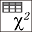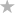#### File Exchange > Data Analysis >    Chi-Square Test for Contingency TableAuthor:
OriginLab Technical Support
1/11/2023
Last Update:
4/27/2023
109
Total Ratings:
0
File Size:
374 KB
Average Rating:File Name:
Chi-Square...le.opx
File Version:
1.00
Minimum Versions:
Type:
App
Summary:

Perform Chi-Square Test for contingency table to determine whether there is significant evidence of association between two variables.

Description:

Purpose

This App can be used to perform Chi-Square Test for contingency table to determine whether there is significant evidence of association between two variables.

Installation

Download the file "Chi-Square Test for Contingency Table.opx", and then drag-and-drop onto the Origin workspace. An icon will appear in the Apps gallery window.

Operation

1. Activate a worksheet with data and click the icon in the Apps Gallery window to open the dialog.
2. Select a column for Group Label and multiple columns for Data Range.
3. The column category can be stored in different column label rows (Long Name, Comment, Paramter etc.).Choose one for Column Category.
4. Click OK button.

Sample OPJU File
This app provides a sample OPJU file.  Right click the App icon in the Apps Gallery window, and choose Show Samples Folder from the short-cut menu. A folder will open. Drag-and-drop the project file Chi-Square Test for Contingency Table Sample.opju from the folder onto Origin. The Notes window in the project shows detailed steps.
Note: If you wish to save the OPJU after changing, it is recommended that you save to a different folder location (e.g. User Files Folder).

Algorithm

The Pearson Chi-Square test statistic: $$Q_p=\sum_{i}^{}\sum_{j}^{}(n_{ij}-e_{ij})^2/e_{ij}$$

The Likelihood Ratio Chi-Square test statistic: $$G^2=2\sum_{i}^{}\sum_{j}^{}n_{ij}ln(n_{ij}/e_{ij})$$

where $$n_{ij}$$ is the observed frequency in table cell (i, j) and $$e_{ij}$$ is the expected frequency for table cell (i, j).

Expected frequency: $$e_{ij}=(n_{i\cdot}n_{\cdot j})/n$$